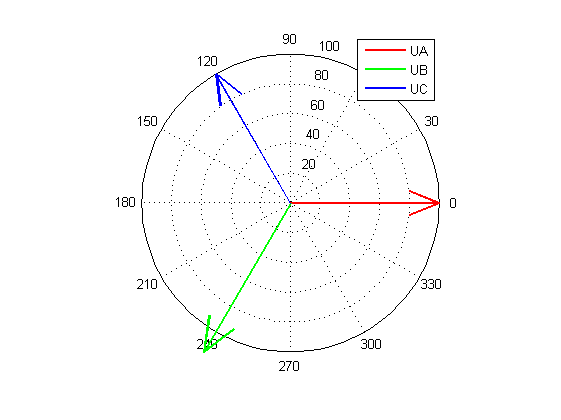# How to Evaluate Voltage Unbalance Using Symmetrical Components Method, and Determine Magnitudes by Known K2U (K0U)

To evaluate voltage unbalance we can use Symmetrical components method proposed by Charles Fortescue. Vector of three phase voltages can be expressed as a sum of three vectors (phasors): zero- positive- and negative-sequence.

$U_{abc} = \begin{bmatrix} U_a \ U_b \ U_c \end{bmatrix} = \begin{bmatrix} U_{a,0} \ U_{b,0} \ U_{c,0} \end{bmatrix} + \begin{bmatrix} U_{a,1} \ U_{b,1} \ U_{c,1} \end{bmatrix} + \begin{bmatrix} U_{a,2} \ U_{b,2} \ U_{c,2} \end{bmatrix}$ $\alpha \equiv e^{\frac{2}{3}\pi i}$ \begin{align} U_{abc} &= \begin{bmatrix} U_0 \ U_0 \ U_0 \end{bmatrix} + \begin{bmatrix} U_1 \ \alpha^2 U_1 \ \alpha U_1 \end{bmatrix} + \begin{bmatrix} U_2 \ \alpha U_2 \ \alpha^2 U_2 \end{bmatrix} = \ \end{align} \begin{align} &= \textbf{A} U_{012} \end{align}

From these phasors we can derive negative-sequence voltage unbalance ratio K2U and zero-sequence voltage unbalance ratio K0U

$K_{2U} = \frac{U_2}{U_1}$ $K_{0U} = \frac{U_0}{U_1}$

Here is MATLAB script that produces the desired level of both negative- and -zero voltage unbalance ratio, then sets respective phasor magnitudes. After that we estimate unbalance using Symmetrical components method and draw a phasor diagramm.To generate signal with known level of unbalance $K_{2U}$ we can change magnitude of one of the voltages in the balanced three phase system. So we set all angles between voltages = 120 deg. Magnitudes of Ua and Uc should be equal (Ua=Uc). Ub should be set as

$U_b = U_a \frac{1+2 K_{2U} }{1-K_{2U}}$

This equation can be easily derived if we notice that

$U_1 K_{2U} = \frac{1}{3} (U_b + 2 U_a) K_{2U} = \frac{1}{3} (U_b - U_a) = U_2$